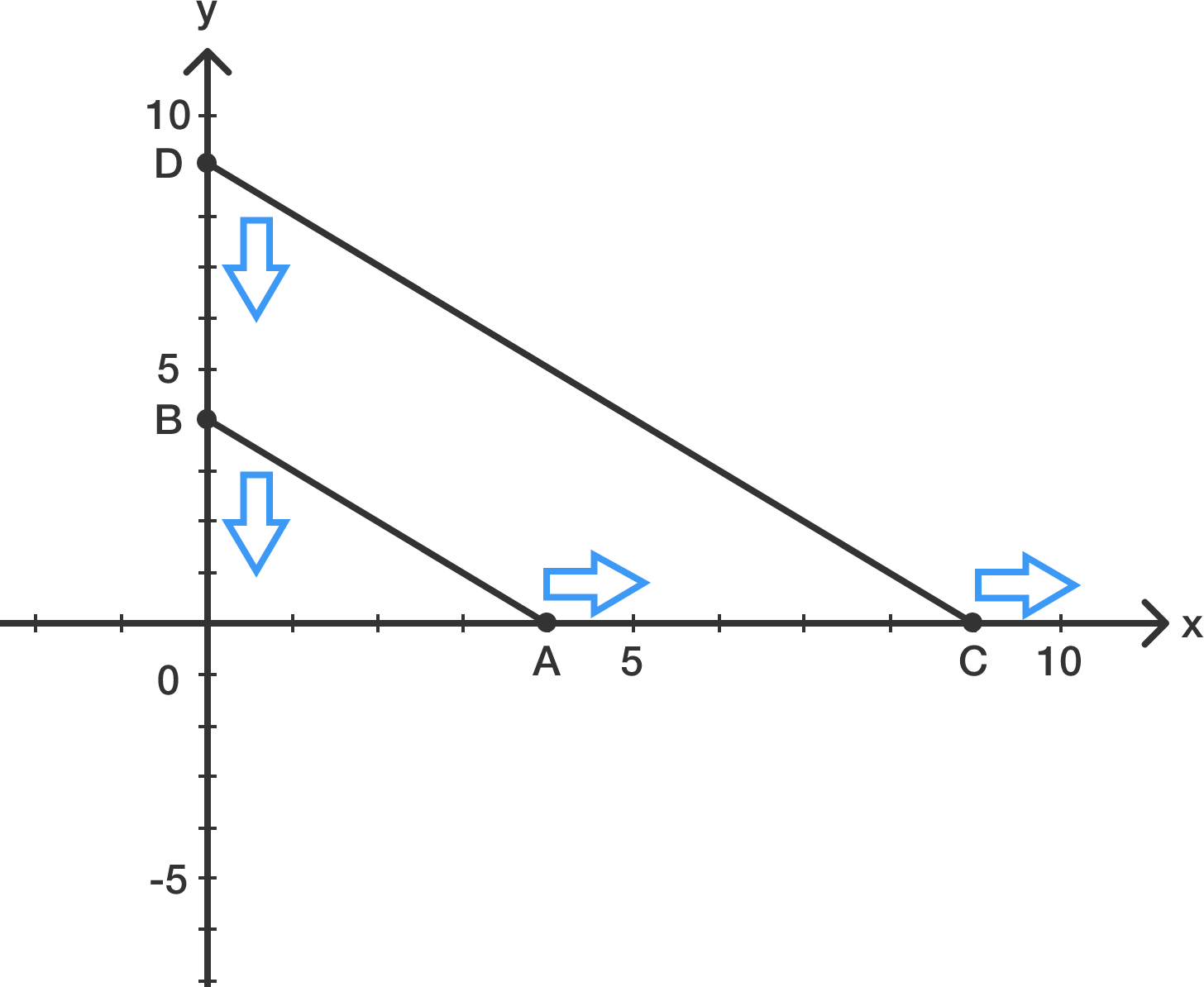# Dancing Rods

Geometry Level 3Let two Rods $AB$ of length $10$ and $CD$ of length $20$ are sliding on smooth standard co-ordinate axis such that their ends are always con-cyclic. If the locus of centre of that circle which pass through all four points $A,B,C,D$ is expressed as:

$(ax-by)^2 + (bx-ay)^2 = c^2$

For positive integers $a,b,c$. What is the minimum value of $a+b+c$

Details and Assumptions

• Diagram not up to scale
###### Inspired from Kushal Patankar's Problem
×

Problem Loading...

Note Loading...

Set Loading...